Example Questions

Example Question #121 : How To Find The Probability Of An Outcome

Khalil has a spinner and a six-sided die. The spinner is divided into five equal sections. The five sections are labeled Red, Green, Blue, Yellow, and Purple, individually. Each side of the die corresponds to a number one through six.

What is the probability of Khalil spinning a primary color and rolling an odd number?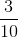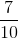Explanation:

Remember that the probability of an event is given by dividing the number of desired outcomes by the number of total outcomes.

The probability of Khalil spinning one of the three primary colors (Red, Blue, or Yellow) isThe probability of Khalil rolling an odd number (1, 3, or 5) is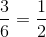We want to know the probability of Khalil spinning a primary color AND rolling an odd number. To do this compound probability, we multiply the two probabilities together.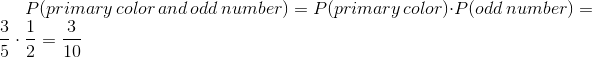Example Question #122 : How To Find The Probability Of An Outcome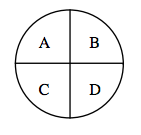The figure above showers a spinner divided into four equal sections.

If Raul spins the spinner, what is the probability that he does NOT spin an A?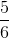None of the given answers are correct.Explanation:

The probability of an event is given by dividing the number of desired outcomes by the number of total outcomes.

In this case, we want to find all the possibilities of Raul spinning anything but an A. That means, he can spin a B, C, or D.

Therefore, there are three desired outcomes out of a total four possible outcomes.

This means that the probability of Raul not spinning an A isExample Question #123 : How To Find The Probability Of An Outcome

Mike is doing a magic trick where two people pull cards without putting them back in the deck. Sam pulls out the Ace of Spades, and Adam pulls out the Queen of Hearts. If Kristi pulls one card, what is the probability that she pulls out an Ace?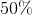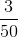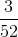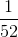Explanation:

If Adam and Sam have already pulled out one card a piece and not put them back in the deck, that means that there are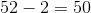cards left.

We also know that one of the Aces has already been pulled. Since there are four Aces in a deck, that means that there areleft.

We know that the probability of an event is the number of desired outcomes divided by the total number of possible outcomes. Therefore, the probability of Kristi pulling an Ace isExample Question #124 : How To Find The Probability Of An Outcome

All the Hearts in a standard deck of playing cards are taken out. Kristen draws one card. What is the probability that she draws a Queen?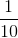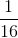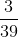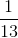Explanation:

A standard deck of cards hascards in it, and it is divided equally into four suits: Hearts, Spades, Clubs, and Diamonds. In this problem, all the Hearts are taken away before Kristen draws her card. That means that our deck has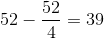total cards in it.

Remember that the probability of an event occurring is expressed as the number of desired outcomes divided by the total number of possible outcomes. In our case, we want to know the probability that Kristen draws a Queen. In our modified deck, there are three Queens (one of Diamonds, Spades, and Clubs), so there are three outcomes that would give us our desired result.

Therefore, the probability that she draws a Queen isExample Question #125 : How To Find The Probability Of An Outcome

Yousef has a spinner that is divided into equal sections. Each section is assigned one specific color: Red, Blue, Green, Orange, and Yellow. Yousef also has two standard six-sided dice.

If he spins the spinner and rolls the two dices, what is the probability that he spins Blue and rolls two sixes?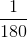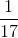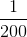Explanation:

Here, we have a compound probability problem. Remember that the probability of a single event is given by dividing the number of desired outcomes by the number of total outcomes.

With that in mind, the probability of Yousef's spinner landing on Blue issince there are section possible outcomes and one desired outcome.

For the first die, the probability of him rolling a six is. The same goes for the second die since the two rolls are independent of one another.

The probability of multiple events happening together can be expressed like this: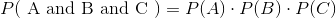Therefore, the probability of Yousef spinning Blue and rolling two sixes is: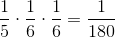Example Question #126 : How To Find The Probability Of An Outcome

Forty balls are placed in a large box, each marked with a whole number from 1 to 40. The balls marked with an even number or a prime number are all blue; the other balls are red.

A ball is drawn and replaced; the process is repeated. Give the probability that a blue ball is drawn both times.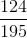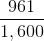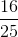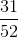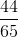Explanation:

There are twenty even whole numbers from 1 to 40 - 2, 4, 6, up to 40. Also, there are eleven odd primes - 3, 5, 7, 11, 13, 17, 19, 23, 29, 31, 37 (there is one even prime, 2, but it has already been counted). Therefore, the box will contain 31 blue balls out of 40, and the probability that the draw of one ball will result in the ball being blue is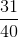. This same probability holds in both the first and second draws.

Since the draws are independent events, the probabilities can be multiplied: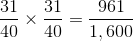,

the correct choice.

Example Question #127 : How To Find The Probability Of An Outcome

Twenty-six balls are placed in a box, each with a different letter of the alphabet. All twenty-six letters are represented.

A ball is drawn, then replaced; the process is repeated two more times. What is the probability that a ball with a vowel is drawn all three times?

(Note: "Y" is to be considered a consonant here.)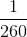None of the other responses gives a correct answer.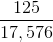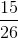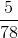Explanation:

5 of the 26 balls are marked with vowels, so the probability that the draw of one ball will result in the ball being marked with a vowel is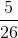; this probability is the same for each of the three draws. Since the draws are independent events, the probabilities can be multiplied: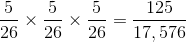.

Example Question #128 : How To Find The Probability Of An Outcome

Balls are placed in a large box. Each ball is marked with a whole number from 1 to 20; each even number is represented by two balls, and each odd number is represented by one ball.

A ball is drawn, then replaced; the process is then repeated. Give the probability that an even number will be drawn both times.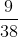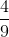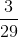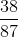Explanation:

There are 10 even numbers and 10 odd numbers in the set of whole numbers from 1 to 20. Therefore, there are 20 even-numbered balls and 10 odd-numbered balls - a total of 30 balls in the box.

The probability that the draw of one ball will result in an even-numbered ball is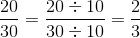This probability is the same for each of the two draws. Since the draws are independent events, the probabilities can be multiplied: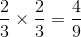,

the correct response.

Example Question #129 : How To Find The Probability Of An Outcome

Balls are placed in a large box. Each ball is marked with a whole number from 1 to 20; each even number is represented by two balls, and each odd number is represented by one ball.

Two balls are drawn without replacement. Give the probability that both balls will be marked with an odd number.None of the other responses gives a correct answer.Explanation:

There are 10 even numbers and 10 odd numbers in the set of whole numbers from 1 to 20. Therefore, there are 20 even-numbered balls and 10 odd-numbered balls - a total of 30 balls in the box.

Assume that the balls are drawn one after the other. The probability that the first ball will be odd is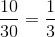.

Since the ball is not replaced, there are now 29 balls total, 9 of which are marked with odd numbers. The probability that the second ball will be odd is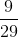.

Multiply these probabilities to get the probability that both balls will be marked with odd numbers: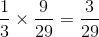.

Example Question #130 : How To Find The Probability Of An Outcome

Twenty-six balls are placed in a box, each with a different letter of the alphabet. All twenty-six letters are represented.

Three balls are drawn without replacement. What is the probability that all three letters on the balls will be consonants?

(Note: "Y" is to be considered a consonant here.)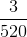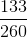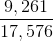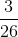None of the other responses gives a correct answer.Explanation:

We can assume that the three balls are drawn one at a time.

When the first ball is drawn, there are 26 balls in the box, 21 of which are marked with consonants. The probability of drawing a ball marked with a consonant is therefore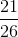.

Since there is no replacement, when the second ball is drawn, there are 25 balls in the box, 20 of which are marked with consonants. The probability of drawing a ball marked with a consonant is therefore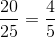.

Similarly, when the third ball is drawn, there are 24 balls in the box, 19 of which are marked with consonants. The probability of drawing a ball marked with a consonant is therefore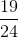.

Multiply the three probabilities together. The probability that all three balls will be marked with consonants is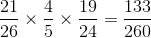.